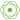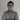# Difference between Mono and Flux

If you’re using Project Reactor, or any of the frameworks that use it, such as Spring WebFlux, or a reactive Spring Data library, you probably encountered the terms Mono and Flux. In this (small) tutorial I’ll explore the differences between them.

### Reactive streams

Project Reactor is a framework open sourced by Pivotal, which acts as a foundation for reactive applications. Nowadays it’s based upon the reactive streams initiative, an initiative founded by engineers from Netflix, Pivotal and Lightbend with contributions from other big Java-players such as Oracle and Red Hat.

Within this initiative, the reactive stream specification was created, with several key factors such as:

• A reactive stream should be non-blocking,
• It should be a stream of data,
• It should work asynchronously,
• And it should be able to handle back pressure.

The reason why is because when we write applications as usual, and we make database calls, HTTP calls, …, we send the request, block the thread until there’s a response, and then continue. While this certainly works, it’s probably a waste of resources.

### The Publisher

This means you no longer can return simple POJO’s, but you have to return something else, something that can provide the result when it’s available. Within the reactive streams initiative, this is called a `Publisher`. A `Publisher` has a `subcribe()` method that will allow the consumer to get the POJO when it’s available.

`Publisher` (for example `Publisher<Foo>`) can return zero or multiple, possibly infinite, results. To make it more clear how many results you can expect, Project Reactor (the reactive streams implementation of Pivotal) introduced two implementations of `Publisher`:

• `Mono<T>`, which can either return zero or one result before completing,
• And a `Flux<T>`, which can return zero to many, possibly infinite, results before completing.

### The Mono

The first type of publisher is a `Mono`. The `Mono` API allows you to emit only a single value, after which it will immediately complete. This means that the `Mono` is the reactive counter-part of returning a simple object, or an `Optional`.

For example, let’s say we have the following code:

``````public Person findCurrentUser() {
if (isAuthenticated()) return new Person("Jane", "Doe");
else return null;
}``````

In Java 8, we could rewrite this as:

``````public Optional<Person> findCurrentUser() {
if (isAuthenticated()) return Optional.of(new Person("Jane", "Doe"));
else return Optional.empty();
}``````

If we’re using reactive streams on the other hand, we would use the following code:

``````public Mono<Person> findCurrentUser() {
if (isAuthenticated()) return Mono.just(new Person("Jane", "Doe"));
else return Mono.empty();
}``````

### The Flux

While the `Mono` is used for handling zero or one result, the `Flux` is used to handle zero to many results, possibly even infinite results. We can see this as the reactive-counter part of a collection, an array or a stream.

For example, if we have the following code:

``````public List<Person> findAll() {
return Arrays.asList(
new Person("Jane", "Doe"),
new Person("John", "Doe")
);
}``````

In Java 8, we could rewrite this by using streams:

``````public Stream<Person> findAll() {
return Stream.of(
new Person("Jane", "Doe"),
new Person("John", "Doe")
);
}``````

And with reactive streams, we can rewrite this as:

``````public Flux<Person> findAll() {
return Flux.just(
new Person("Jane", "Doe"),
new Person("John", "Doe")
);
}``````

As you probably can tell, the concepts of reactive streams overlap with the concepts we’ve seen with functional programming. That’s why Venkat Subramaniam also calls it functional programming++.

### The subscriber

Let’s assume the following code, what will be printed on the console:

``````Flux
.just(1, 2, 3, 4)
.reduce(Integer::sum)
.log();``````

The answer to that question would be, nothing, nothing at all. Reactive streams use a push model. This means that items are pushed on the stream at the pace of the publisher, regardless of whether the subscriber can follow or not (no worries, backpressure is a key-feature of reactive streams).

By that logic, one would think that the reactive stream in the previous example would emit the 10. However, reactive streams are lazy, and won’t start as long as there is no subscriber present. That means that a subscriber is always necessary with reactive streams.

### Asynchronous nature

I’ve mentioned before that publishers are asynchronous in nature, but are they always asynchronous? The answer to that is, no, not always. Whether or not a reactive stream is synchronous or asynchronous, depends on the type of publisher you use.

For example, let’s assume the following code:

``````AtomicInteger sum = new AtomicInteger(0);
Flux
.just(1, 2, 3, 4)
.reduce(Integer::sum)
.subscribe(sum::set);
log.info("Sum is: {}", sum.get());``````

What do you think will happen? Will it print 10 on the console, because that’s the sum of those numbers, or will it print 0 on the console, because the stream is handled asynchronously, and thus the result hasn’t been calculated yet when it reaches the logging statement?

The answer to that question is that it will print 10 onto the console, because `Flux.just()` by default uses the current thread, and thus the result has been calculated when it reaches the logging statement.

Now, what would be the result in the following example?

``````AtomicInteger sum = new AtomicInteger(0);
Flux
.just(1, 2, 3, 4)
.subscribeOn(Schedulers.elastic())
.reduce(Integer::sum)
.subscribe(sum::set);
logger.info("Sum is: {}", sum.get());``````

The answer in this case would be 0, because using `subscribeOn()` will execute the subscription on a different scheduler worker thread, making it asynchronous.

So, depending on the nature of the reactive stream, it will be either synchronous or asynchronous. Code like I just wrote is a bad practice when you work with reactive streams. The proper solution would have been:

``````Flux
.just(1, 2, 3, 4)
.reduce(Integer::sum)
.susbcribe(sum -> logger.info("Sum is: {}", sum);``````

With Project Reactor, there are already a lot of built-in publishers. However, in some cases you’ll have to create your own publishers. Luckily, both `Mono` and `Flux` come with their own `create()` method, that provides you a sink to properly emit items on.

For example, let’s say we want to use the Twitter4J library with reactive streams, in that case, we could write:

``````return Flux.create(sink -> {
});``````

This is also an example of an infinite stream, as the amount of tweets will go on forever (or until Twitter shuts down).

### Hot and cold streams

If you worked with a reactive library like RxJS, you’re probably familiar with the terms hot and cold observables. The difference between them is that if you use multiple subscribers to a cold observable, the source is restarted, while for a hot observable, the same source is used for both subscribers.

By default, streams in project reactor are cold. We can demonstrate that using the following code:

``````Flux<Integer> numbers = Flux
.just(1, 2, 3, 4)
.log();
numbers
.reduce(Integer::sum)
.subscribe(sum -> logger.info("Sum is: {}", sum));
numbers
.reduce((a, b) -> a * b)
.subscribe(product -> logger.info("Product is: {}", product));``````

In this example, the numbers 1 to 4 are emitted twice, once for the first subscriber, and another time for the second subscriber.

However, in some cases, like executing a HTTP request, you don’t want to start from the source again, and you want to turn your cold stream into a hot one. Within Project Reactor, we can do that by sharing:

``````Flux<Integer> numbers = Flux
.just(1, 2, 3, 4)
.log()
.share();
numbers
.reduce(Integer::sum)
.subscribe(sum -> logger.info("Sum is: {}", sum));
numbers
.reduce((a, b) -> a * b)
.subscribe(product -> logger.info("Product is: {}", product));``````

By doing this, the values 1 to 4 are emitted only once, and the same source is shared for both subscribers.

For `Mono`, we don’t have a `share()` method, so we should use the `cache()` method in stead. This turns it into something similar as a `BehaviorSubject` within RxJS, where the value is cached and emitted for every new subscriber.

With that, we’ve seen the basics of reactive streams and the differences between `Mono` and `Flux`.# 👋 Hey there, I'm Dimitri

I'm a full-stack developer who likes testing out new and interesting frameworks and blogging about them. I prefer working with Java and JavaScript.